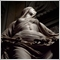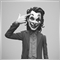# iHighest and iLowest functions not working as I'd like them to.21

Hello there,

I'm trying to build an EA based on breakouts. I want it to open a buy order whenever the price is above the 30 day high and the RSI is below 70, and a sell order whenever the price is below the 30 day low and the RSI is above 30. This is the code that I've written for it, but for some reason, it does not work.

```void Entry()
{
double DImas =  iADX(Symbol(), Timeframe, Periodo, PRICE_CLOSE, MODE_PLUSDI, 0);
double DImenos =  iADX(Symbol(), Timeframe, Periodo, PRICE_CLOSE, MODE_MINUSDI, 0);
double RSI = iRSI(Symbol(), 60, Periodo, PRICE_CLOSE, 0);
int Max = iHighest(Symbol(), 60, MODE_CLOSE, 30, 0);
int Min = iLowest(Symbol(), 60, MODE_CLOSE, 30, 0);
double MaxVal = High[Max];
double MinVal = Low[Min];
{
if (DImas > DImenos)
{
if(Close > MaxVal)
{
}
}
if (DImenos > DImas)
{
if(Close < MinVal)
{
if (RSI > 30) Sell();
}
}
}```

It does what I tell it, but it also sends orders when there's no breakout of the 30 day extrema.

Any idea on what's going on?16538

Nephelion:

Hello there,

I'm trying to build an EA based on breakouts. I want it to open a buy order whenever the price is above the 30 day high and the RSI is below 70, and a sell order whenever the price is below the 30 day low and the RSI is above 30. This is the code that I've written for it, but for some reason, it does not work.

This never happen.

```void Entry()
{
double DImas =  iADX(Symbol(), Timeframe, Periodo, PRICE_CLOSE, MODE_PLUSDI, 0);
double DImenos =  iADX(Symbol(), Timeframe, Periodo, PRICE_CLOSE, MODE_MINUSDI, 0);
double RSI = iRSI(Symbol(), 60, Periodo, PRICE_CLOSE, 0);
int Max = iHighest(Symbol(), 60, MODE_CLOSE, 30, 0);
int Min = iLowest(Symbol(), 60, MODE_CLOSE, 30, 0);
double MaxVal = High[Max];
double MinVal = Low[Min];
{
if (DImas > DImenos)
{
if(Close > MaxVal)
{
}
}
if (DImenos > DImas)
{
if(Close < MinVal)
{
if (RSI > 30) Sell();
}
}
}```21

This never happen.

So I have to set the shift = 1 on both functions?626

30 day low or high indices are given by

```int Max = iHighest(Symbol(), PERIOD_D1, MODE_CLOSE, 30, 0);
int Min = iLowest(Symbol(), PERIOD_D1, MODE_CLOSE, 30, 0);```20233

```     double DImenos =  iADX(Symbol(), Timeframe, Periodo, PRICE_CLOSE, MODE_MINUSDI, 0);
double RSI = iRSI(Symbol(), 60, Periodo, PRICE_CLOSE, 0);```
In addition, On MT4: Unless the current chart is that specific pair/TF referenced, you must handle 4066/4073 errors.